# SSAT Middle Level Math : How to find the area of a rectangle

## Example Questions

### Example Question #61 : Quadrilaterals

The area of a rectangle with a length of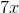and a width of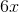is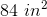. Find.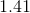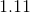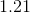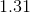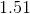Explanation:

The area of a rectangle is the product of length and width: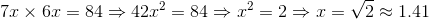### Example Question #21 : How To Find The Area Of A Rectangle

Give the area of a rectangle with a length of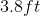and a width of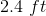.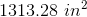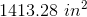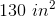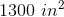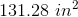Explanation:

We know that: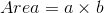where: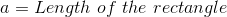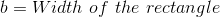So we can write: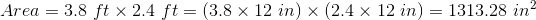### Example Question #22 : How To Find The Area Of A Rectangle

A rectangular table has a length of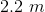and a width of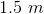. Give the area of the table.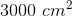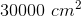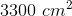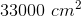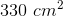Explanation:

We know that:where:So we can write: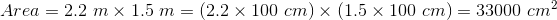### Example Question #23 : How To Find The Area Of A Rectangle

The length of a rectangle is three times longer than its width. If the width of the rectangle ismeters, give the area of the rectangle.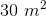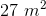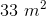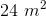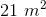Explanation:

The length of the rectangle would be: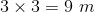We know that:where:So we can write: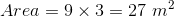### Example Question #24 : How To Find The Area Of A Rectangle

Which of the following is the area of a rectangle with a width of 4 feet and a length that is twice the width?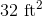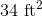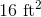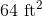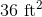Explanation:

The area of a rectangle is found by multiplying the width by the length.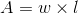We know that the width is 4 feet and the the length must be twice the width. Multiply the width by 2 to find the length.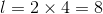Multiply the length and width to find the area.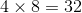### Example Question #25 : How To Find The Area Of A Rectangle

Jeff decided to build a play area for his guinea pigs. The play area would be an enclosure 6 feet long, 2 feet wide, and 2 feet tall. In cubic feet, how big is the play area?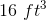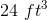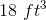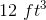Explanation:

The cubic feet of an area is found by multiping the length times the width times the height. Given that the length is six feet, the width is two feet, and that the height is two feet, the total cubic area would be found using this equation: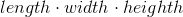Here is the equation with the appropriate numbers plugged in: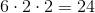Therefore, 24 cubic feet is the correct answer.

### Example Question #6 : Geometry

Jessica's blanket is 12 square feet. Lisa has a blanket that is half the size of Jessica's blanket. Which of the following are possible dimensions of Lisa's blanket?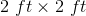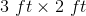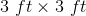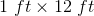Explanation:

The area of a rectangle if found by multiplying the length times the width. Here, we know that Lisa's blanket is half the area of Jessica's blanket. Since Jessica's blanket is 12 square feet, that means that Lisa's blanket must be 6 square feet.

The only length and width values that give us 6 square feet when multiplied by one another are 3 feet by 2 feet. This is therefore the correct answer.

### Example Question #21 : How To Find The Area Of A Rectangle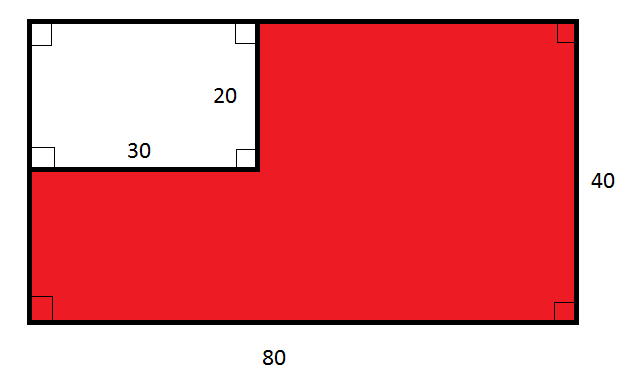Note: Figure NOT drawn to scale.

What percent of the above figure is red?

The correct answer is not given among the other choices.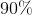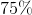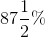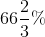The correct answer is not given among the other choices.

Explanation:

The large rectangle has length 80 and width 40, and, consequently, area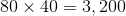.

The white region is a rectangle with length 30 and width 20, and, consequently, area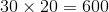.

The red region, therefore, has area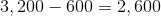The red region is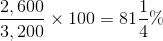of the large rectangle.

This is not one of the choices.

### Example Question #27 : How To Find The Area Of A RectangleNote: Figure NOT drawn to scale.

Refer to the above diagram. Give the ratio of the area of the red region to that of the white region.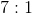The correct answer is not given among the other choices.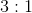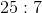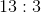Explanation:

The large rectangle has length 80 and width 40, and, consequently, area.

The white region is a rectangle with length 30 and width 20, and, consequently, area.

The red region, therefore, has areaThe ratio of the area of the red region to that of the white region is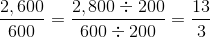That is, 13 to 3.

### Example Question #28 : How To Find The Area Of A Rectangle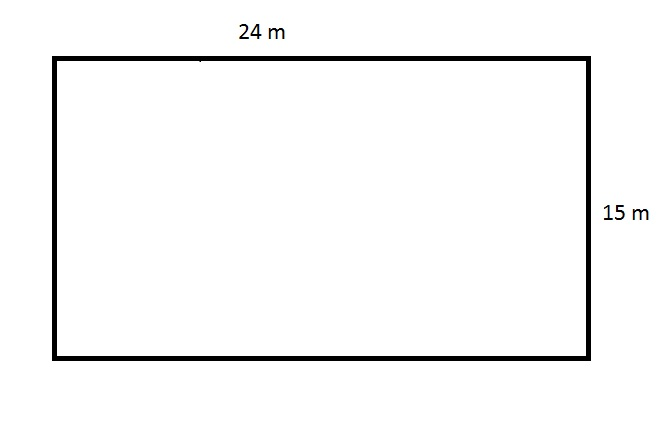The above figure depicts the rectangular swimming pool at an apartment. The apartment manager needs to purchase a tarp that will cover this pool completely, but the store will only sell the material in multiples of one hundred square meters. How many square meters will the manager need to buy?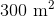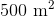Insufficient information is given to answer the question.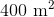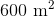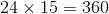square meters.